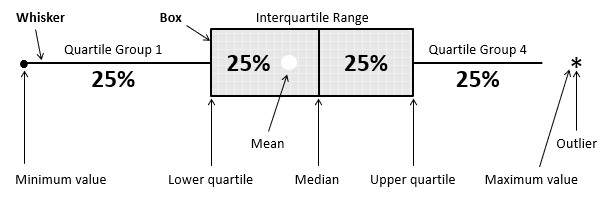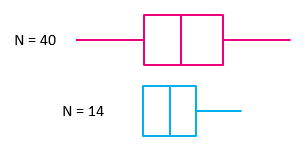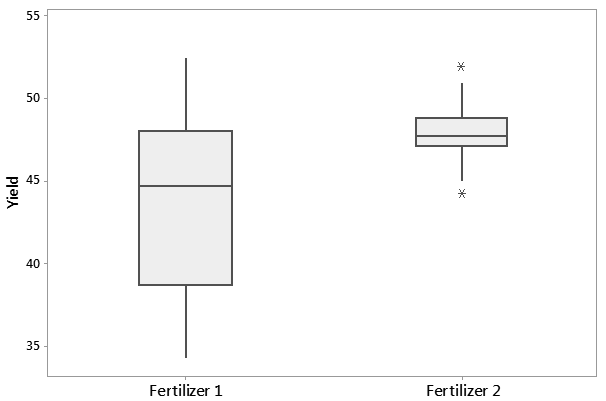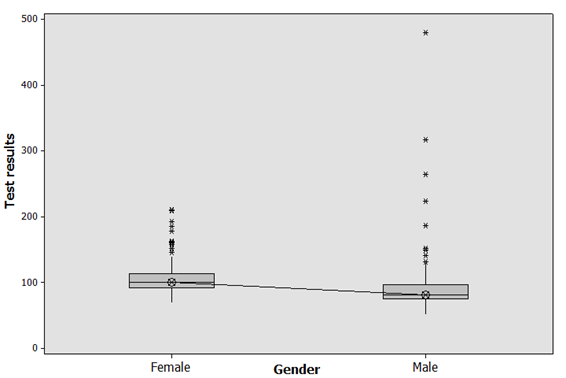By the CIToolkit content team   |   3 minutes read

# Box Plotbox plot is a graph that shows the frequency of numeric data values. It can be drawn either horizontally or vertically. It is referred to as a Box-and-Whisker Plot as it displays the data in a box-and-whiskers format. Box plots are widely used in statistics, scientific research, higher education, process improvement, and in social and human sciences.

Box plots are mainly used to explore data as well as to present the data in an easy and understandable manner. They are similar to histograms and provide similar information but in different graphical formats. They provide a quick way for examining the central tendency, the amount of variation in the data, as well as the presence of gaps, outliers or unusual data points.A box plot may show key statistics such as the median of the data, maximum and minimum values, as well as the lower and upper quartiles. The data is plotted in such away that the bottom 25% and the top 25% of the data points are represented by the two whiskers, whereas the middle 50% of the data points are represented by the box.

Box plots are most useful when comparing between several data sets. They allow you to compare the central tendency as well as the variability of multiple data sets. They are less detailed than histograms, and take up less space which make them easier to compare. More advanced statistical tests can then be used to test the significance of the differences in terms of central tendency and variability.Like histograms, box plots are ideal to represent moderate to large amount of data. The size of the box plot can vary significantly if the data size is small. Individual value plots are preferred over box plots when representing small amount of data. Box plots can also tell if the distribution is symmetrical or skewed. In a symmetric distribution, the mean and median are nearly the same, and the two whiskers has almost the same length.

## Example

The following are box plots that display the yield of a crop after applying two different fertilizers. Fertilizer 2 appears to have a higher yield than Fertilizer 1.The above chart shows the results of a data set that belongs to Minitab Inc.

What other comments would you make about the above box plots? Think about the variation as well as the presence of any unusual values.

## Example

The below box plot illustrates an analysis that was conducted for diagnosing the presence of diabetes at a workplace.It is evident that females have in general higher glucose levels than males. ANOVA can be used here to test the significance of the difference between the two means.

There are many tools that can help you to create box plots. One of the simplest ways is to use this template.

## Further Information

There are many applications and online services that allow the creation of box plots quickly and automatically (such as Minitab).

Other Formats

Related Articles

Related Templates